## Summary and examples

A quadratic equation has the following general form: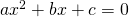Of course we have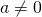, because otherwise the equation would not be quadratic but linear.

In Quadratic equations (factorizing) we have explained under which conditions we can solve a quadratic equation by factorizing. The advantage of that method is that it may provide a solution rapidly. However, this method is not always possible and also requires some skills.

If you do not have the skills or if you do not want to apply this method there is another approach which always works and which is widely known as the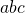-formula. Usually this requires more calculations. On the other hand a solution is guaranteed.

Solving a quadratic equation gives two (real) solutions at most: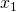or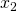: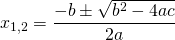Here we have used the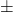notation, but we can also write: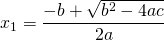or: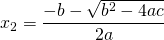We already mentioned that there are at most two solutions. This depends on the value of the discriminant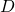, i.e. the formula under the root notation: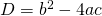We distinguish three cases:

1.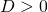In this case the solutionsandare different and then we say that the equation has two solutions.

2.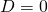In this case the solutionsandare equal and then we say that the equation has just one solution (actually 2 coinciding solutions).

3.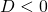In this case the equation has no real solutions and then we say that the equation has no solutions.
We give a number of examples.

##### Example 1

Solve: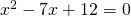We notice immediately that the left-hand side can be factorized: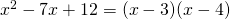and the equation: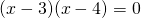has the following solution: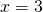of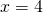For comparison, we also solve this equation with the-formula: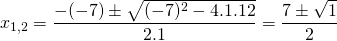and we find, as expected:ofWhen do we use the-formula? When you have enough skills to try the factorizing method you would prefer this although you know this will not always help you. If this method does not give the results sufficiently fast, then you would apply the-formula.

##### Example 2

Solve: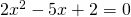In this case it is not easy to use factorizing (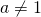and if you would divide both sides byyou get fractions as coefficients). So we have to apply the-formula: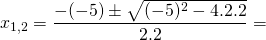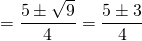and find the following solutions: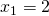or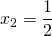##### Example 3

Solve: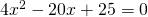Applying the-formula we get: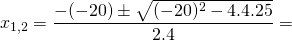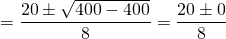The solution is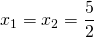. The equation has two coinciding solutions, sometimes it is said that the equation has just one solution.

Note that the solution can also be found by applying the following special product:Applying this formula in the equation above we notice that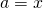en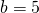. Then we can write: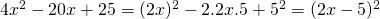##### Example 4

Solve: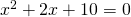If we apply the-formule we immediately notice that: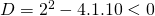Thus the equation has no solution.

##### Example 5

Solve: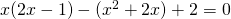This equation does not have the standard general form and has to be rewritten. We remove the parentheses: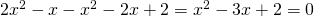and factorize the result: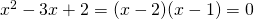The solutions are: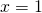or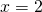The-formula provides the same result.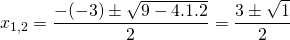0